Publicité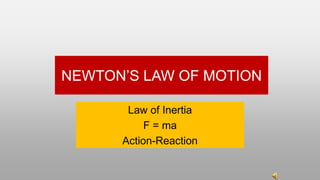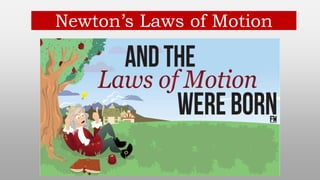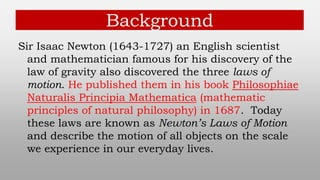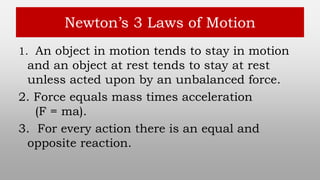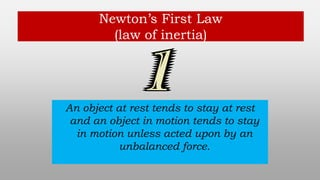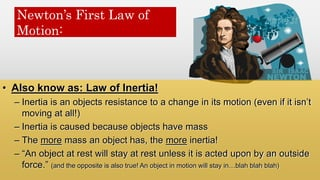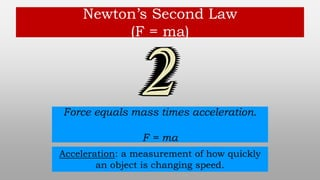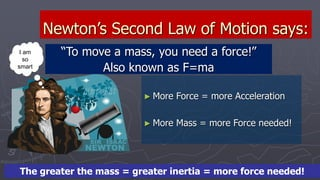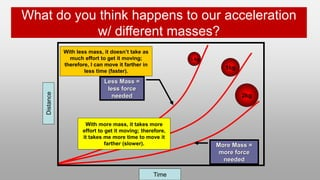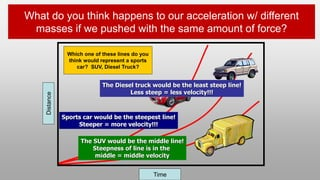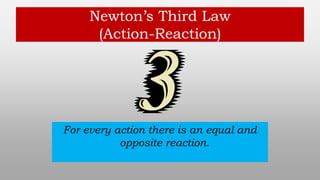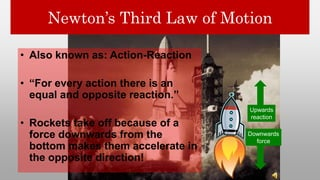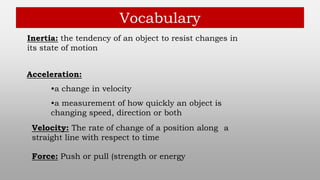1 sur 13
Publicité

### Newtons-Laws.pptx

1. NEWTON’S LAW OF MOTION Law of Inertia F = ma Action-Reaction
2. Newton’s Laws of Motion
3. Background Sir Isaac Newton (1643-1727) an English scientist and mathematician famous for his discovery of the law of gravity also discovered the three laws of motion. He published them in his book Philosophiae Naturalis Principia Mathematica (mathematic principles of natural philosophy) in 1687. Today these laws are known as Newton’s Laws of Motion and describe the motion of all objects on the scale we experience in our everyday lives.
4. Newton’s 3 Laws of Motion 1. An object in motion tends to stay in motion and an object at rest tends to stay at rest unless acted upon by an unbalanced force. 2. Force equals mass times acceleration (F = ma). 3. For every action there is an equal and opposite reaction.
5. Newton’s First Law (law of inertia) An object at rest tends to stay at rest and an object in motion tends to stay in motion unless acted upon by an unbalanced force.
6. Newton’s First Law of Motion: • Also know as: Law of Inertia! – Inertia is an objects resistance to a change in its motion (even if it isn’t moving at all!) – Inertia is caused because objects have mass – The more mass an object has, the more inertia! – “An object at rest will stay at rest unless it is acted upon by an outside force.” (and the opposite is also true! An object in motion will stay in…blah blah blah)
7. Newton’s Second Law (F = ma) Force equals mass times acceleration. F = ma Acceleration: a measurement of how quickly an object is changing speed.
8. ► More Force = more Acceleration ► More Mass = more Force needed! Newton’s Second Law of Motion says: “To move a mass, you need a force!” Also known as F=ma I am so smart … The greater the mass = greater inertia = more force needed!
9. What do you think happens to our acceleration w/ different masses? Distance Time Less Mass = less force needed More Mass = more force needed ½ kg 1kg 2kg With less mass, it doesn’t take as much effort to get it moving; therefore, I can move it farther in less time (faster). With more mass, it takes more effort to get it moving; therefore, it takes me more time to move it farther (slower).
10. What do you think happens to our acceleration w/ different masses if we pushed with the same amount of force? Distance Time Which one of these lines do you think would represent a sports car? SUV, Diesel Truck? Sports car would be the steepest line! Steeper = more velocity!!! The Diesel truck would be the least steep line! Less steep = less velocity!!! The SUV would be the middle line! Steepness of line is in the middle = middle velocity
11. Newton’s Third Law (Action-Reaction) For every action there is an equal and opposite reaction.
12. Newton’s Third Law of Motion • Also known as: Action-Reaction • “For every action there is an equal and opposite reaction.” • Rockets take off because of a force downwards from the bottom makes them accelerate in the opposite direction! Downwards force Upwards reaction
13. Vocabulary Inertia: the tendency of an object to resist changes in its state of motion Acceleration: •a change in velocity •a measurement of how quickly an object is changing speed, direction or both Velocity: The rate of change of a position along a straight line with respect to time Force: Push or pull (strength or energy
Publicité